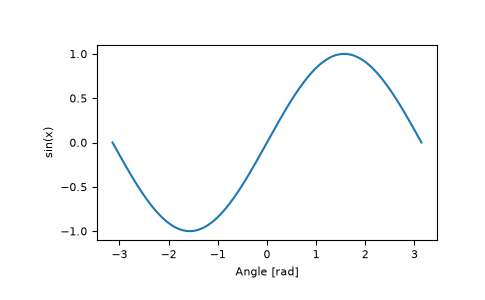# numpy.sin¶

numpy.sin(x, /, out=None, *, where=True, casting='same_kind', order='K', dtype=None, subok=True[, signature, extobj]) = <ufunc 'sin'>

Trigonometric sine, element-wise.

Parameters
xarray_like

Angle, in radians ($$2 \pi$$ rad equals 360 degrees).

outndarray, None, or tuple of ndarray and None, optional

A location into which the result is stored. If provided, it must have a shape that the inputs broadcast to. If not provided or None, a freshly-allocated array is returned. A tuple (possible only as a keyword argument) must have length equal to the number of outputs.

wherearray_like, optional

This condition is broadcast over the input. At locations where the condition is True, the out array will be set to the ufunc result. Elsewhere, the out array will retain its original value. Note that if an uninitialized out array is created via the default out=None, locations within it where the condition is False will remain uninitialized.

**kwargs

For other keyword-only arguments, see the ufunc docs.

Returns
yarray_like

The sine of each element of x. This is a scalar if x is a scalar.

Notes

The sine is one of the fundamental functions of trigonometry (the mathematical study of triangles). Consider a circle of radius 1 centered on the origin. A ray comes in from the $$+x$$ axis, makes an angle at the origin (measured counter-clockwise from that axis), and departs from the origin. The $$y$$ coordinate of the outgoing ray’s intersection with the unit circle is the sine of that angle. It ranges from -1 for $$x=3\pi / 2$$ to +1 for $$\pi / 2.$$ The function has zeroes where the angle is a multiple of $$\pi$$. Sines of angles between $$\pi$$ and $$2\pi$$ are negative. The numerous properties of the sine and related functions are included in any standard trigonometry text.

Examples

Print sine of one angle:

>>> np.sin(np.pi/2.)
1.0


Print sines of an array of angles given in degrees:

>>> np.sin(np.array((0., 30., 45., 60., 90.)) * np.pi / 180. )
array([ 0.        ,  0.5       ,  0.70710678,  0.8660254 ,  1.        ])


Plot the sine function:

>>> import matplotlib.pylab as plt
>>> x = np.linspace(-np.pi, np.pi, 201)
>>> plt.plot(x, np.sin(x))